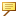Rules for Using Relations
Consider the following rules:
If you try to assign a relation outside the section to a parameter that is already driven by a section relation, you get an error message when the system regenerates the model. The same applies if you try to assign a relation in a section to a parameter that is already driven by a relation outside the section. Remove one of the relations and regenerate model.
If an assembly tries to assign a value to a dimension variable that is already driven by a part or subassembly relation, you get an error message. Remove one of the relations and regenerate the model.
Modifying the units of a model can invalidate your relations, as they are not scaled along with the model.
The relations are evaluated from top to bottom. After executing the relations, the final values of the parameters are locked. However, if a parameter value is based on a condition, the parameter is locked only if the condition remains true after executing all the relations.
The parameter can be locked due to usage of relations as follows:
If a parameter is used in relation unconditionally, for example, MYPARAM = 5.
If a parameter is used in conditional relations, the parameter is locked if at least one of the conditions is true at the end of execution of the relations.The locked parameter value can be different from the value assigned in the condition.
In the following relations:
A = 0
IF a==0
b=5
endif
if a ==5
b = 10
endif
a = 5
The parameter B is locked, if A==5 is true at the end of execution, but the value of B will be 5 during executing, if A==5 is false.In some cases, relations defined at different levels may conflict. Check the validity of relations by clickingand make sure your relations fulfill your design intent.